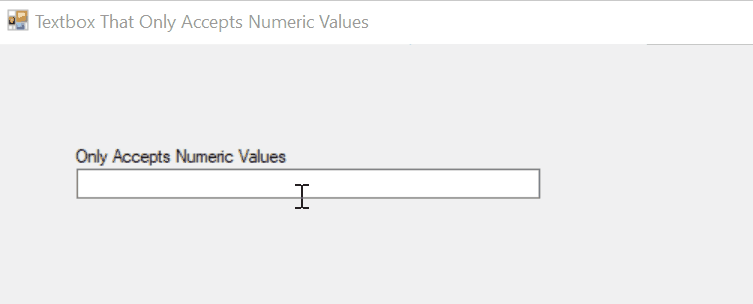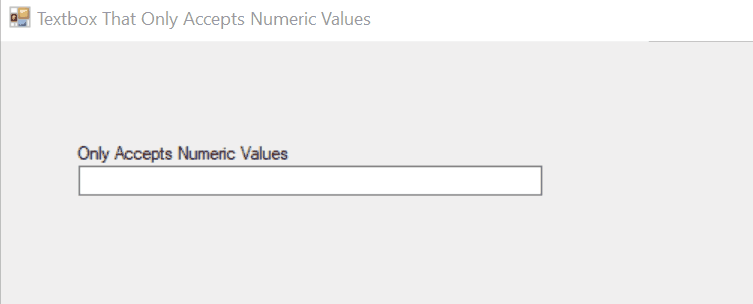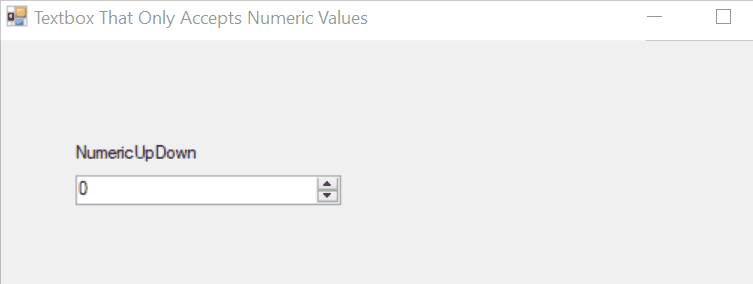# C# 新建一個僅接受數字的文字框

## 使用 C# 中的 KeyPressEventArgs 類建立一個僅接受數字的文字框

`KeyPressEventArgs` 是一個 C# 類，用於指定使用者輸入的字元按下一個鍵。它的 `KeyChar` 屬性返回使用者鍵入的字元。在這裡，我們使用了 `KeyPress` 事件將文字框限制為僅數字值。

``````!char.IsControl(e.KeyChar) && !char.IsDigit(e.KeyChar) &&
(e.KeyChar != '.');
``````

``````using System;
using System.Collections.Generic;
using System.ComponentModel;
using System.Data;
using System.Drawing;
using System.Linq;
using System.Text;
using System.Windows.Forms;
namespace WindowsFormsApplication1
{
public partial class Form1 : Form
{
public Form1()
{
InitializeComponent();
}
private void textBox1_KeyPress(object sender, KeyPressEventArgs e)
{
if (!char.IsControl(e.KeyChar) && !char.IsDigit(e.KeyChar) &&
(e.KeyChar != '.'))
{
e.Handled = true;
}

// only allow one decimal point
if ((e.KeyChar == '.') && ((sender as TextBox).Text.IndexOf('.') > -1))
{
e.Handled = true;
}
}
}
}
``````

``````//only allows numeric values in the textbox
``````## 使用 C# 中的 `Regex.IsMatch()` 方法建立一個僅接受數字的文字框 `Textbox`

``````Regex.IsMatch(StringName, @"Expression");
``````

``````using System;
using System.Collections.Generic;
using System.ComponentModel;
using System.Data;
using System.Drawing;
using System.Linq;
using System.Text;
using System.Windows.Forms;
namespace WindowsFormsApplication1
{
public partial class Form1 : Form
{
public Form1()
{
InitializeComponent();
}
private void textBox1_TextChanged(object sender, EventArgs e)
{
if (System.Text.RegularExpressions.Regex.IsMatch(textBox1.Text, "  ^ [0-9]"))
{
textBox1.Text = "";
}
}

}
}
``````

``````//A textbox created that only accepts numbers.
``````## 使用 `NumericUpDown` 方法建立一個僅接受數字的文字框 `Textbox`

`NumericUpDown` 為使用者提供了使用文字框提供的向上和向下按鈕輸入數值的介面。你可以簡單地從工具箱中拖放 `NumericUpDown` 來建立僅接受數字的文字框。

``````private System.Windows.Forms.NumericUpDown numericUpDown;
this.numericUpDown = new System.Windows.Forms.NumericUpDown();
``````## 相關文章 - Csharp Textbox

• 在 C# 中將佔位符新增到文字框